•matlab
• 1.3 牛顿割线法 1.4 史蒂芬森迭代法 1.5 确定有根区间 2.MATLAB代码 2.1 主函数 2.2 二分法函数 2.3 牛顿迭代法函数 2.4 牛顿割线法函数 2.5 史蒂芬森迭代法函数 2.6 所求方程原函数及一阶导形式 3.运行...

目录
1.理论铺垫
1.1 二分法
1.2 牛顿迭代法
1.3 牛顿割线法
1.4 史蒂芬森迭代法
1.5 确定有根区间 <
展开全文二分法 MATLAB解方程
•matlab
• 数值计算(二分法、简单迭代法、Newton迭代法、弦截法(割线法、双点弦法)).doc文档
• 文档内容为数值分析算法的C++实现。 算法包括：非线性方程求解、高斯消元发、高斯列主消元法、牛顿迭代法、割线法
• 牛顿迭代法、双点割线法及改进的双点割线法的比较1 题目：求=0的根。2 算法组织：2.1 牛顿迭代法算法原理见《计算方法教程（第2版）》，凌永祥、陈明奎编著，西安交通大学出版社，2005年4月第2版，第194至第195页。...
牛顿迭代法、双点割线法及改进的双点割线法的比较
1 题目：
求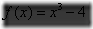=0的根。
2 算法组织：
2.1 牛顿迭代法
算法原理见《计算方法教程（第2版）》，凌永祥、陈明奎编著，西安交通大学出版社，2005年4月第2版，第194至第195页。
代码：（C代码，运行环境为VC++6.0）
//牛顿迭代法解非线性方程
//---------------------Include Files-------------
#include
#include
//-----------------end Include Files-------------
#define M 10//迭代次数
double f(double x);//f(x)函数
double df(double x);//f(x)的导数
int main()
{
double x;
int i = 1;
x = 1;
for(; i < M; i++)
{
x[i] = x[i-1] - f(x[i-1]) / df(x[i-1]);
printf("x[%d] = %.7lf/n", i, x[i]);
}
return(0);
}
double f(double x)
{
return  (pow(x,3)-4);
}
double df(double x)
{
return (3*pow(x,2));
}
2.2 双点割线法
算法原理见《计算方法教程（第2版）》，凌永祥、陈明奎编著，西安交通大学出版社，2005年4月第2版，第197至第199页。
代码：（C代码，运行环境为VC++6.0）
//双点割线法
//---------------------Include Files-------------
#include
#include
//-----------------end Include Files-------------
#define M 10//迭代次数
double f(double x);//f(x)函数
int main()
{
double x;
int i = 1;
double s = 0;
x = 0;
x = 1.0;
for(; i < M; i++)
{
s = f(x[i]) / f(x[i-1]);
x[i+1] = x[i] + s * ( x[i] - x[i-1] ) / ( 1 - s );//(5-11c)式
printf("x[%d] = %.7lf/n", i+1, x[i+1]);
}
return(0);
}
double f(double x)
{
return  (pow(x,3)-4);
}
2.3 改进的双点割线法
改进之处主要在2.2部分的代码中加了一个条件判断，算法原理见《计算方法教程（第2版）》，凌永祥、陈明奎编著，西安交通大学出版社，2005年4月第2版，第199页SECANT1算法。
代码：（C代码，运行环境为VC++6.0）
//改进的双点割线法
//---------------------Include Files-------------
#include
#include
//-----------------end Include Files-------------
#define M 10//迭代次数
double f(double x);//f(x)函数
int main()
{
int i = 1;
double s = 0;
double f0, f1, x0, x1, f_temp, x_temp;
x0 = 1.0;
x1 = 2.0;
f0 = f(x0);
f1 = f(x1);
if(fabs(f1) > fabs(f0))//改进的地方
{
x_temp = x0;
x0 = x1;
x1 = x_temp;
x_temp = f0;
f0 = f1;
f1 = x_temp;
}
for(; i < M; i++)
{
s = f1/ f0;
x_temp = x1 - s * ( x0 - x1 ) / ( 1 - s );//(5-11c)式
printf("x[%d] = %.7lf/n", i+1, x_temp);
f_temp = f(x_temp);
if(fabs(f_temp)>fabs(f1))//改进的地方
{
x0 = x_temp;
f0 = f_temp;
}
else
{
x0 = x1;
f0 = f1;
x1 = x_temp;
f1 = f_temp;
}
}
return(0);
}
double f(double x)
{
return (pow(x,3)-4);
}
3 计算结果与分析：
3.1 计算结果：
Newton迭代法计算=0的解，取x0 = 1.0
x = 2.0000000
x = 1.6666667
x = 1.5911111
x = 1.5874097
x = 1.5874011
x = 1.5874011
x = 1.5874011
x = 1.5874011
x = 1.5874011
Press any key to continue
Newton迭代法计算=0的解，取x0 = 2.0
x = 1.6666667
x = 1.5911111
x = 1.5874097
x = 1.5874011
x = 1.5874011
x = 1.5874011
x = 1.5874011
x = 1.5874011
x = 1.5874011
Press any key to continue
用双点割线法计算=0的解：取x0 = 1.0, x1 = 2.0
x = 1.4285714
x = 1.5504587
x = 1.5914243
x = 1.5873061
x = 1.5874008
x = 1.5874011
x = 1.5874011
x = 1.5874011
x = 1.5874011
Press any key to continue
用改进后的双点割线法（加上|f1|>|f0|的判断条件）计算=0的解：取x0 = 1.0, x1 = 2.0
x = 1.4285714
x = 1.6712329
x = 1.5787586
x = 1.5869588
x = 1.5874035
x = 1.5874011
x = 1.5874011
x = 1.5874011
x = 1.5874011
Press any key to continue
3.2 结果分析，f(1) < 0, f(2) > 0, 所以(1, 2)间必有f(x) = 0的解。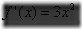，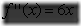在牛顿迭代法中，取x0 = 1.0，不满足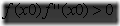这个条件，但牛顿迭代仍收敛。说明第212页的定理5.4中并不能包含所有的牛顿迭代收敛情况，即，满足定理 5.4的牛顿迭代必定收敛，但不满足定理5.4的牛顿迭代并不一定不收敛，也可能收敛。
另外，发现取x0 = 2.0时，满足，即满足定理5.4，收敛速度比取x0 = 1.0时要快。
改进的双点割线法并没有比普通双点割线法的收敛速度快。
展开全文include 算法 c
• 分别用二分法、试位法、不动点迭代、Newton-Raphson法和割线法求解并比较各方法的收敛速度


二分法、试位法、不动点迭代法、牛顿法、割线法
问题回顾问题分析1.二分法2.试位法3.不动点迭代4.Newton-Raphson法5.割线法小结

问题回顾
一段质量均匀分布的电缆线悬挂在两点之间，构成一段悬链，其满足如下微分方程：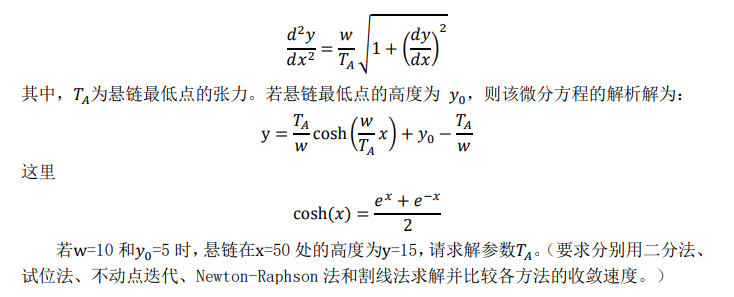问题分析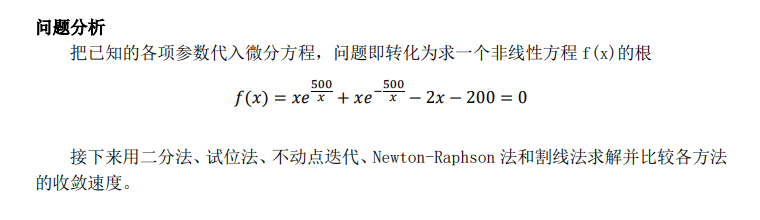1.二分法
首先确定有根区间，将区间二等分，通过判断f(x)的符号，逐步将有根区间缩小，直至有根区间足够地小，便可求出满足精度要求的近似根。通过悬链最低点张力的唯一性，可以确定该区间只有一个根。
用 (xl,xr)来表示我们所感兴趣的根，初始为(1000,1500)，MATLAB脚本程序如下，保留10位有效数字。
clear;
x_l = 1000; % the initial value of the left bound of x is 1000
x_r = 1500; % the initial value of the right bound of x is 15000
x_m = mean([x_l,x_r]); % x_m is the mean value of the two bounds
x_g = 0; % x_g is the target value of the Bolzano method
e_a = 5e-11;
i = 0;
if f(x_l)*f(x_r)>0
error('invalid input');
else
while (abs(x_g-x_m)/x_m>e_a) % tolerance of accuracy
if f(x_m)==0                 % find the accurate root
break;
else
if f(x_l)*f(x_m)<0
x_r = x_m;               % update the new estimation to the interval
else
x_l = x_m;
end
end
x_g = x_m;
x_m = mean([x_r,x_l]);
i = i + 1;
end
end
fprintf('x = %.17f  i = %d\n', x_m, i);

程序输出为
x = 1266.32436044747009873 i = 32
本例中用了 32 次迭代达到了保留10位有效数字的计算准则。下面用图表示出迭代过程。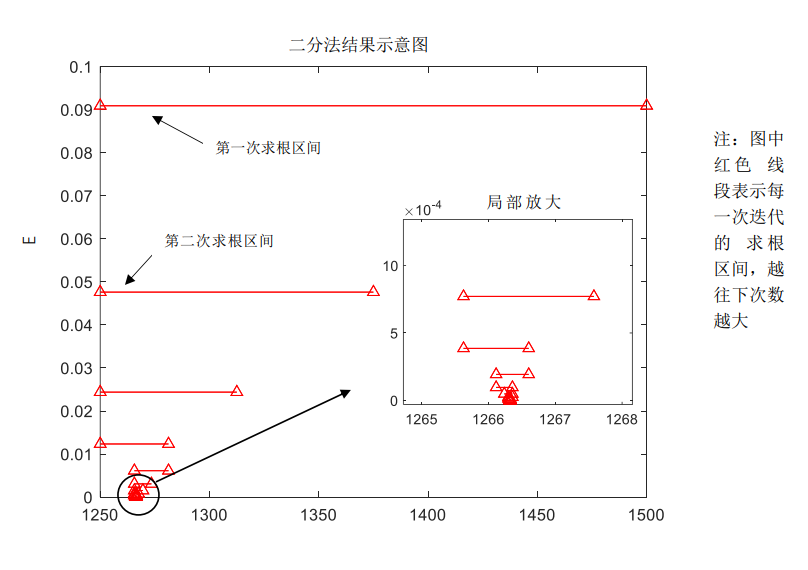2.试位法
与二分法不同，每次区间的划分点不在中点，而是两区间函数值点连线与 x 轴的交点， 递归地做这样的划分求解最终的结果，图示结果附后。
clear;
x_l = 1000; % the initial value of the left bound of x is 1000
x_r = 1500; % the initial value of the right bound of x is1500
x_c = x_r; % x_c is the cross point of the two bound and the x-axis
x_t = 0; % x_t is the target ROOT of the eqation
e_a = 5e-11;
i = 0; % iteration tag
while (abs(x_t-x_c)/x_c>e_a)
x_t=x_c; % update the target value with the cross point
if f(x_t)==0
break;
else
x_c = x_r - f(x_r)*(x_l-x_r)/(f(x_l)-f(x_r));
if f(x_c)*f(x_r)<0
x_l = x_c;
else
x_r = x_c;
end
end
i = i+1;
end
fprintf('x = %.17f  i = %d\n', x_c, i);

程序输出为
x = 1266.32436040640868669 i = 16
本例中用了 16 次迭代达到了保留10位有效数字的计算准则。下面用图表示出迭代过程。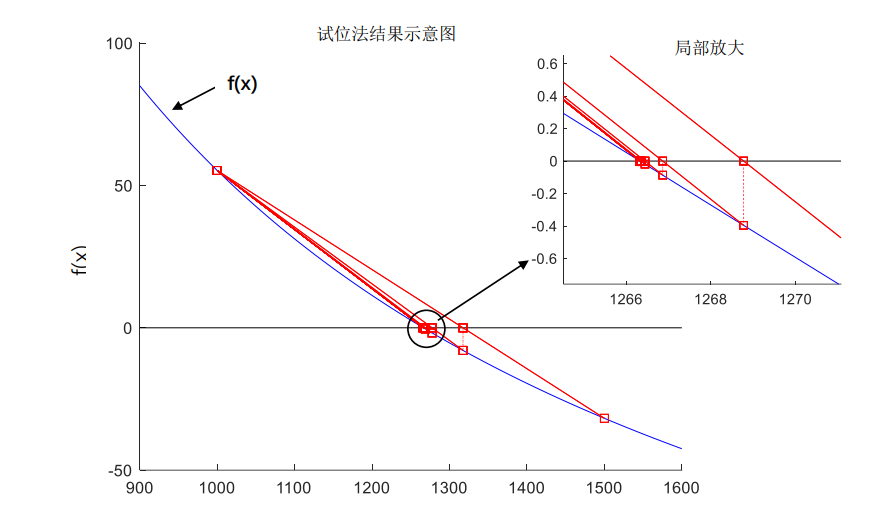根据计算结果可以看到，此例中试位法较二分法更为快速地收敛到了想要的解，且并也已经出现“一个划界点保持不动”的现象。
3.不动点迭代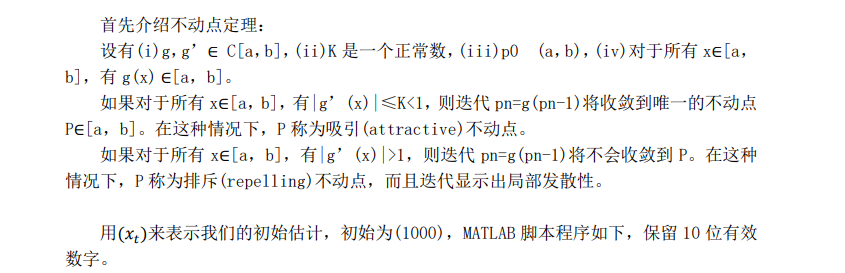clear;
x_t = 1000;	% the initial is 1000
x_c = 0;
e_a = 5e-11;
for i=1:1000  		 % iteration tag
x_c = f(x_t) + x_t;
if(abs(x_c-x_t)/x_c<e_a) % tolerance of accuracy
break;
end
x_t = x_c;
end
fprintf('x = %.17f  i = %d\n', x_c, i);

程序输出为
x = 1266.32436009672028376 i = 115
本例中用了 115 次迭代达到了小数点后 10 位有效数字的计算准则。下面用图表示出迭代过程。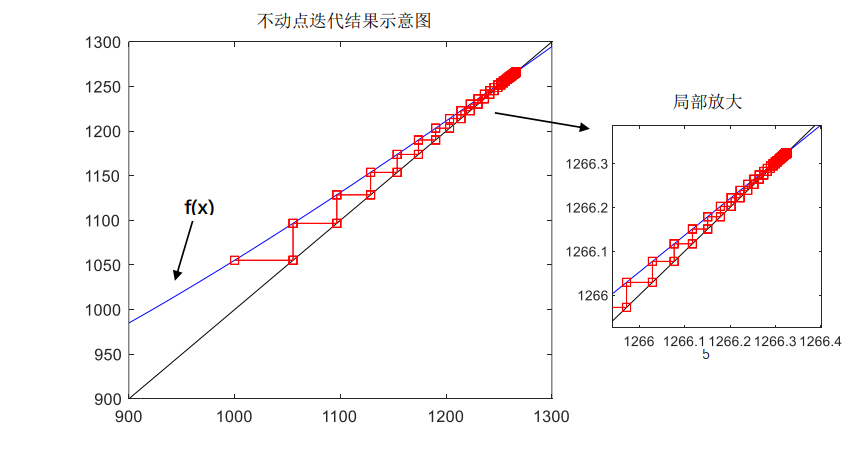4.Newton-Raphson法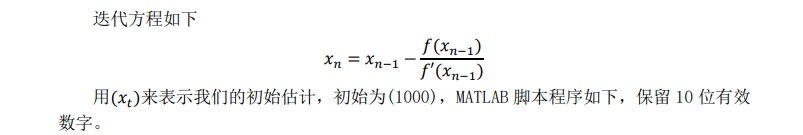clear;
x_t = 1000; % the initial is 1000
x_c = 0;
e_a = 5e-11;
for i=1:1000	% iteration tag
x_c = x_t-f(x_t)/df(x_t);
if(abs(x_c-x_t)/x_c<e_a) % tolerance of accuracy
break;
end
x_t = x_c;
end
fprintf('x = %.17f  i = %d\n', x_c, i);

程序输出为
x = 1266.32436039988829179 i = 5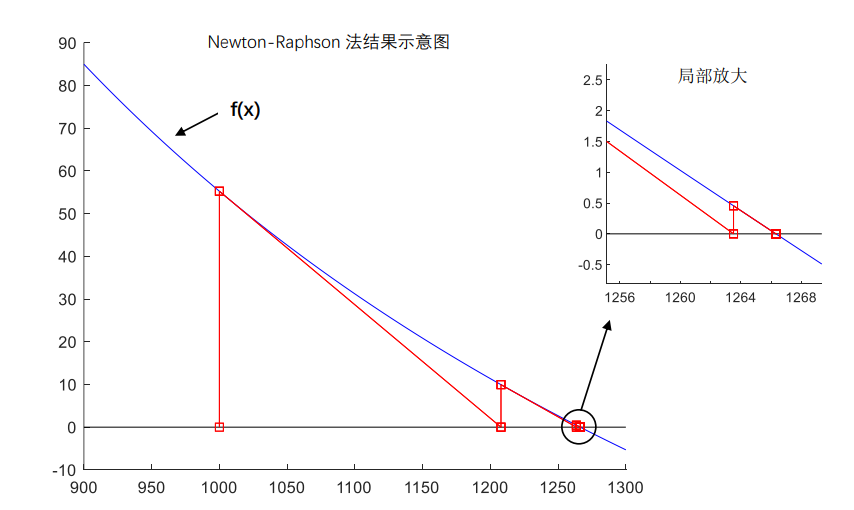5.割线法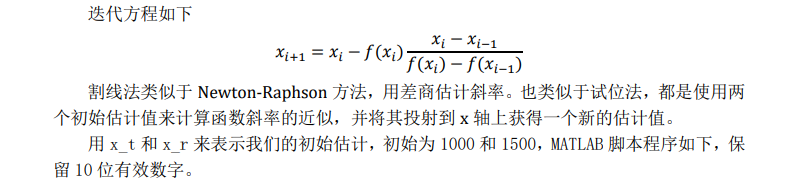clear;
x_t = 1000; % the initial value of the second bound of x is 1000
x_r = 1500; % the initial value of the first bound of x is 1500
x_c = 0;
e_a = 5e-11;
i = 0; % iteration tag
while (abs(x_r-x_t)/x_t>e_a)
x_c=x_t-f(x_t)*(x_t-x_r)/(f(x_t)-f(x_r)); % update the target
if f(x_c)==0
break;
else
x_r = x_t;
x_t = x_c;
end
i = i+1;
end
fprintf('x = %.17f  i = %d\n', x_c, i);

程序输出为
x = 1266.32436039988601806 i = 7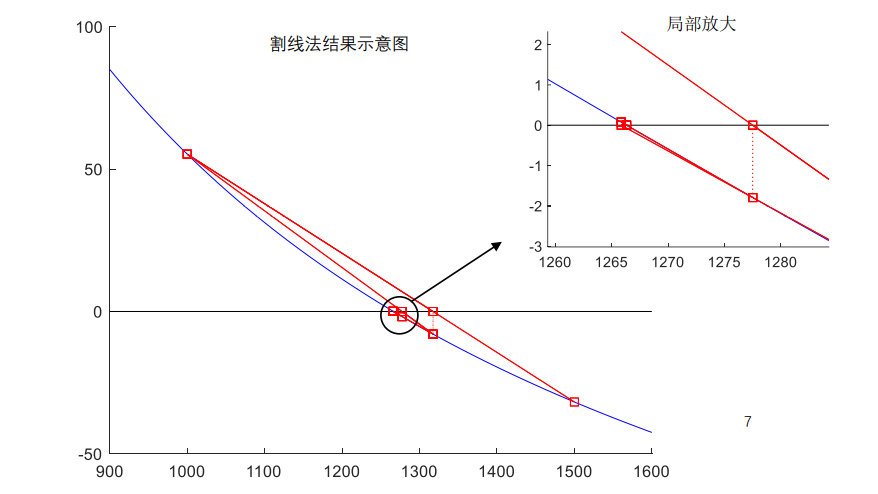小结
本文由实际问题出发，按照题目要求的五种方法分别解了一个非线性的方程，作图比较了五种方法的收敛速度，并用每种方法都得到了最终的结果（有十位有效数字），x = 1266.324360，即悬链最低点的张力为1266.324360。
下面列表总结一下每种方法得到结果的情况：
方法名称初始估计 1初始估计 2迭代次数二分法1000150032试位法1000150016不动点迭代1000\115Newton-Raphson法1000\5割线法100015007
二分法是最简单的方法，且易于在计算机上实现，对函数的条件要求也很低，只要求在有根区间上连续。缺点就是收敛速度较慢，本次实验进行了32次迭代得到结果，与公比为1/2的等比数列收敛速度相同。因此一般不单独使用，常用来为其他方法提供初值区间。
试位法作为二分法的改进，并不是单一的二分区间，而是利用区间两个端点的线性插值来求一个近似根，因此在大多数情况下优于二分法，收敛速度相对于二分法更快。但在实际计算中，如果区间内一阶导数值突然增长，函数图像有极大拐弯，且方程的根更靠近导数大的一侧，情况将会变得非常糟糕，出现“一个划界点保持不动”的现象，此时收敛速度会慢于二分法。本题中没有出现上述情况，进行了16次迭代后得到了结果。
为了减轻上述情况带来的影响，可以对试位法做出如下改进。如果一个端点重复两次或更多次作为新的含根区间的端点，我们将这个点的函数值乘以一个大于0小于1的常数因子w，比如可以取0.5，将停滞的边界点处的函数值变为原来的一半，能够使线性插值的根更接近于精确根。更加符合“试位”的思想。
不动点迭代法与前两种方法不同，只需要一个初始估计值。这种方法收敛较慢，本次实验迭代了115次才得出了最终结果。对初值的要求较高，需要提供较为近似的初始值x0.大多数情况下需要考虑迭代法的局部收敛性，在方成根的附近取初值。不动点迭代法是迭代法的基础，是一种一般性的方法，对研究方程解的存在性、唯一性和具体计算有重要的理论与实用价值。
Newton-Raphson法是经不动点迭代法变形而来的，是迭代法“收敛性”意义上的最优进化。牛顿法的速度很快，本次实验只用了5次迭代就得到了满足要求的解。它的最大优点是方程在单根附近有较高的收敛速度，且算法逻辑简单。但是由于牛顿法是局部收敛的，对初始估计的要求很高，如果没有估计合适，将会产生很差的结果，甚至无法得出答案。此外，牛顿法的每次迭代除了要计算函数值以外，还需要计算导数值，在导数较为复杂时，该方法会比较麻烦。
割线法是牛顿法和试位法的改进。类似于牛顿法，都是用差商估计斜率，但使用了两点的割线，避开了复杂的导数运算。采用两点投射到x轴上得到新的估计值的方法与试位法较为相似，但是初始估计不用界定根。收敛速度在中等到快，本次实验经过7次迭代后得到了结果。
对初始估计的要求很高，如果没有估计合适，将会产生很差的结果，甚至无法得出答案。此外，牛顿法的每次迭代除了要计算函数值以外，还需要计算导数值，在导数较为复杂时，该方法会比较麻烦。
割线法是牛顿法和试位法的改进。类似于牛顿法，都是用差商估计斜率，但使用了两点的割线，避开了复杂的导数运算。采用两点投射到x轴上得到新的估计值的方法与试位法较为相似，但是初始估计不用界定根。收敛速度在中等到快，本次实验经过7次迭代后得到了结果。
总而言之，不同的求解方法各有千秋，我们需要结合实际问题来选择恰当的方法。
展开全文算法 matlab
• #include<stdio.h> #include<math.h> double F(double x) { return cos(x) - x; } double F_(double x) { return -1 - sin(x); } double Newton(double x0,double e,int N) ... x = x.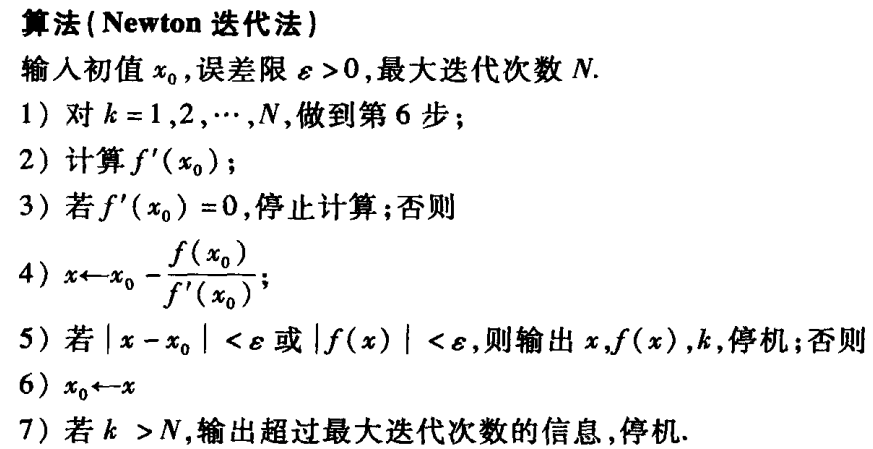#include<stdio.h>
#include<math.h>

double F(double x)
{
return cos(x) - x;
}

double F_(double x)
{
return -1 - sin(x);
}

double Newton(double x0,double e,int N)
{
double x;
int k = 1;
do
{
if (F_(x0) == 0)
break;
else
x = x0 - (F(x0) / F_(x0));
if (fabs(x - x0) < e || fabs(F(x)) < e)
return x;
else
x0 = x;
k++;
} while (k <= N);
return -1;
}

int main()
{
int N = 100;
double x0 = 0, e = 0.00001;
x0 = Newton(x0, e, N);
printf("%lf", x0);
getchar();
}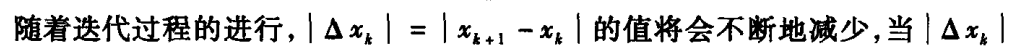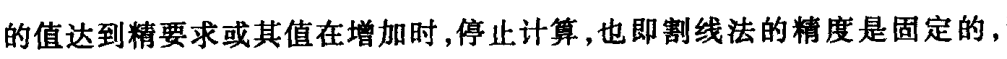#include<stdio.h>
#include<math.h>

double F(double x)
{
return cos(x) - x;
}

double X(double x_k1, double x_k0)
{

return x_k1 - F(x_k1)*((x_k1 - x_k0) / (F(x_k1) - F(x_k0)));
}

double Secant(double x0, double x1, double e)
{
double temp;
while (fabs(x0 - x1) > e)
{
temp = X(x1, x0);
x0 = x1;
x1 = temp;
}
return x1;
}

int main()
{
double x0 = 0, x1 = 1, e = 0.0000001;
x1 = Secant(x0, x1, e);
printf("%lf", x1);
getchar();
}

展开全文• 1.二分法 function y=erfenf(x) y=x.^3-x-x; end a=1; b=1.5; tol=10^(-2); N=10^4; k=0; L=erfenf(a); R=erfenf(b); x=(a+b)/2; y=erfenf(x); while abs(y)>tol&k<N k=k+1; if y*L<... ...matlab 二分法
• 二分法、牛顿法、割线法、简易牛顿法、史蒂芬孙迭代法Matlab代码，经过本人调试已经完美运行，大家可以下载直接用，也可以自己增删内容，都是可以的。
• MATLAB牛顿（Newton）迭代法和割线法求解方程 MATLAB牛顿（Newton）迭代法和割线法求解方程
• 课堂笔记整理：方程求根-二分法、牛顿法、割线法。内容来自周善贵老师的《计算物理》课程。二分法数学基础：对于连续函数 构成的方程： ，如果在区间 上满足： ，则区间 内至少存在一点 ，使得 。基本思想：取区间...
• % 迭代法 二分法 割线法 % (1)【单变量】非线性方程求解 % 函数的调用格式为: % x=fzero(filename,x0) % 其中，filename是待求根方程左端的函数表达式，x0是初始值。 % eg 求 f(x)=x-1/x+5 在 x0=-5 和 x0=1 作为...
• 不动点迭代 function xc = fpi( g, x0, tol ) x(1) = x0; i = 1; while 1 x(i + 1) = g(x(i)); if(abs(x(i+1) - x(i)) < tol) break end i = i + 1; end......